# 9.6.3. Inverting ZTEM Data¶

Here, we invert synthetic ZTEM data using E3DMT versions 1 and 2. When Tipper data, the E3DMT codes have a tendency to place conductive artifacts proximal to the receivers. To overcome this obstacle, we demonstrate a basic approach for limiting artifacts through the use of interface weights.

Important

The ZTEM data are in GIF format; see GIF data conventions page. This means the locations are Easting-Northing-Elevation. The data convention is defined X = Northing, Y = Easting and Z = Down. Failure to recognize this will cause incorrect interpretation of the data. To get addition background information on the coordinate systems used by the E3DMT codes, see the theory sections of the version 1 and version 2 manuals.

## 9.6.3.1. Setup for the Exercise¶

If you have completed the tutorial “Importing, Interpreting and Preparing NSEM Data”:

If you have NOT completed the previous tutorial and would like to start here, complete the following steps:

Pro tip: To avoid confusion between location and data coordinate systems, use the set data headers tool to define location columns as Easting, Northing and Elevation.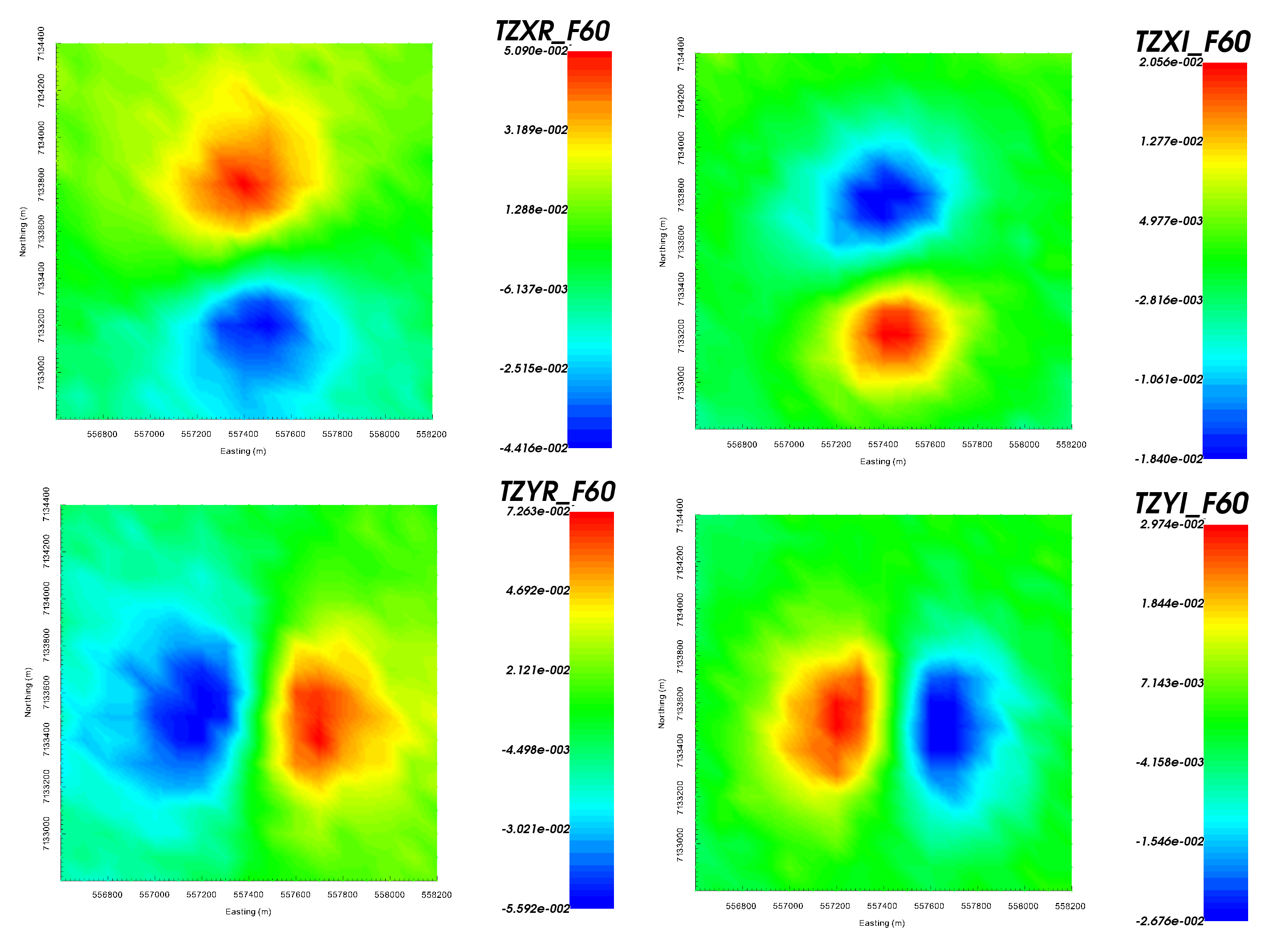Z-axis tipper measurements at 60 Hz. It is important to note that the ‘X’ in TZXR data refers to the Northing direction! ‘R’ refers to real component and ‘I’ refers to imaginary component.

Important

Data were generated using E3DMT version 2 and a block model approximating TKC. A table containing the uncertainties added to the data can be found here .

## 9.6.3.2. E3DMT Version 1¶

Let us now invert the ZTEM data using E3DMT version 1.

• Create E3DMT ver 1 inversion object

• Use edit options to set the inversion parameters

• Basic Tab:
• Select the ZTEM data

• Set mesh

• Set topography to active cells model

• No background susceptibility

• 1D conductivity of 0.001 S/m

• Use Iterative solver unless you have sufficient RAM to use Direct solver.

• Model Options Tab:
• Set Beta cooling schedule to ‘custom by clicking button’. Use beta max = 0.8, beta min = 1e-8 and reduction factor = 0.25

• Set Chi Factor = 0.5 since E3DMT version 1 uses a non-standard measure of data misfit; see manual

• alpha S = 1e-10, alpha E = 1, alpha N = 1 and alpha Z = 2.56 (to recover smoothest model and balance gradient terms based on cell dimensions)

• Set the active cells topo as the active model cells

• Set initial model as 0.001 S/m

• Set reference model as 0.001 S/m

• Set role in model objective function to SMOOTH_MOD_DIF

• Click Apply and write files

• Run the inversion

• View convergence

The results of the inversion are shown below. We are unable to recover the moderately conductive overburden as ZTEM data are not sensitive to layered structures. Despite only inverting data at 2 frequencies, we are generally able to recover the shape of the pipe. The maximum conductivity of the recovered structure is notably higher than the true conductivity of the pipe.

The inversion reaches target misfit after 4 iterations. The model norm is discontinuous because the current model is set to be the reference model for the next beta value. The recovered model is able to reproduce the observed anomaly but underestimates its amplitude.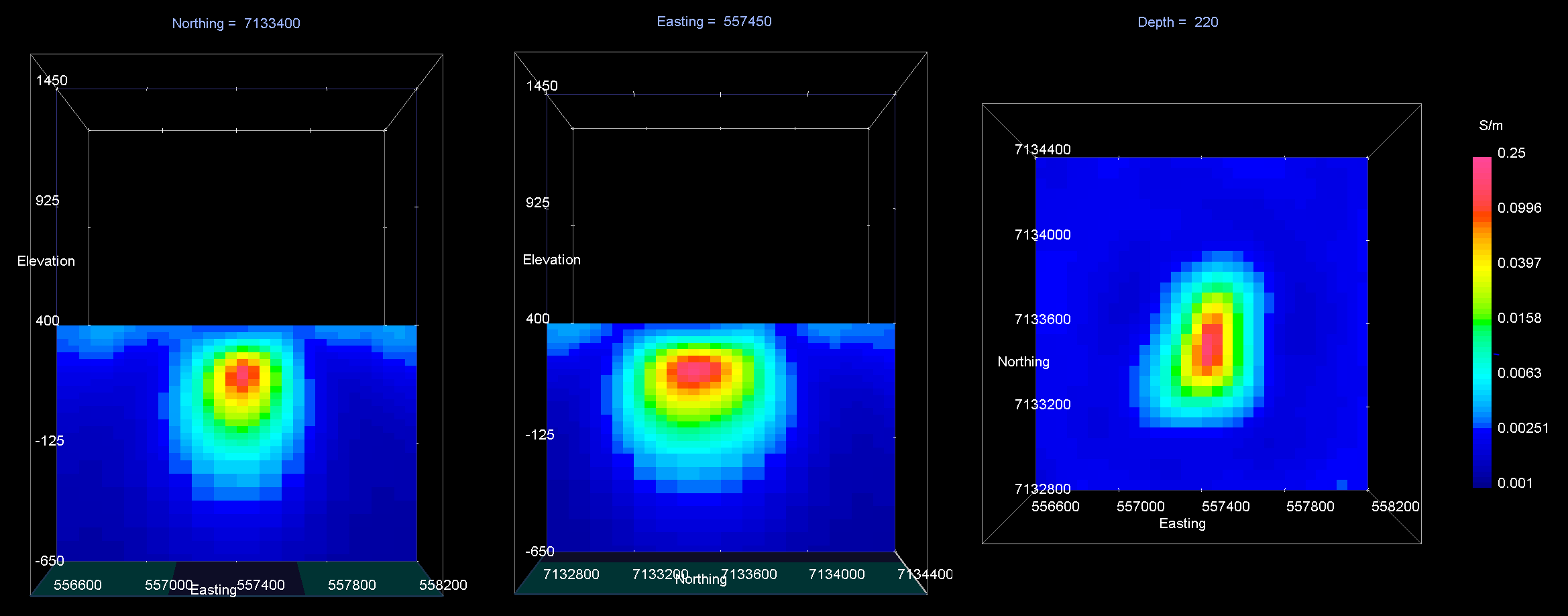Recovered model at iteration 4. EW cross-section (left), NS cross-section (centre), horizontal slice (right).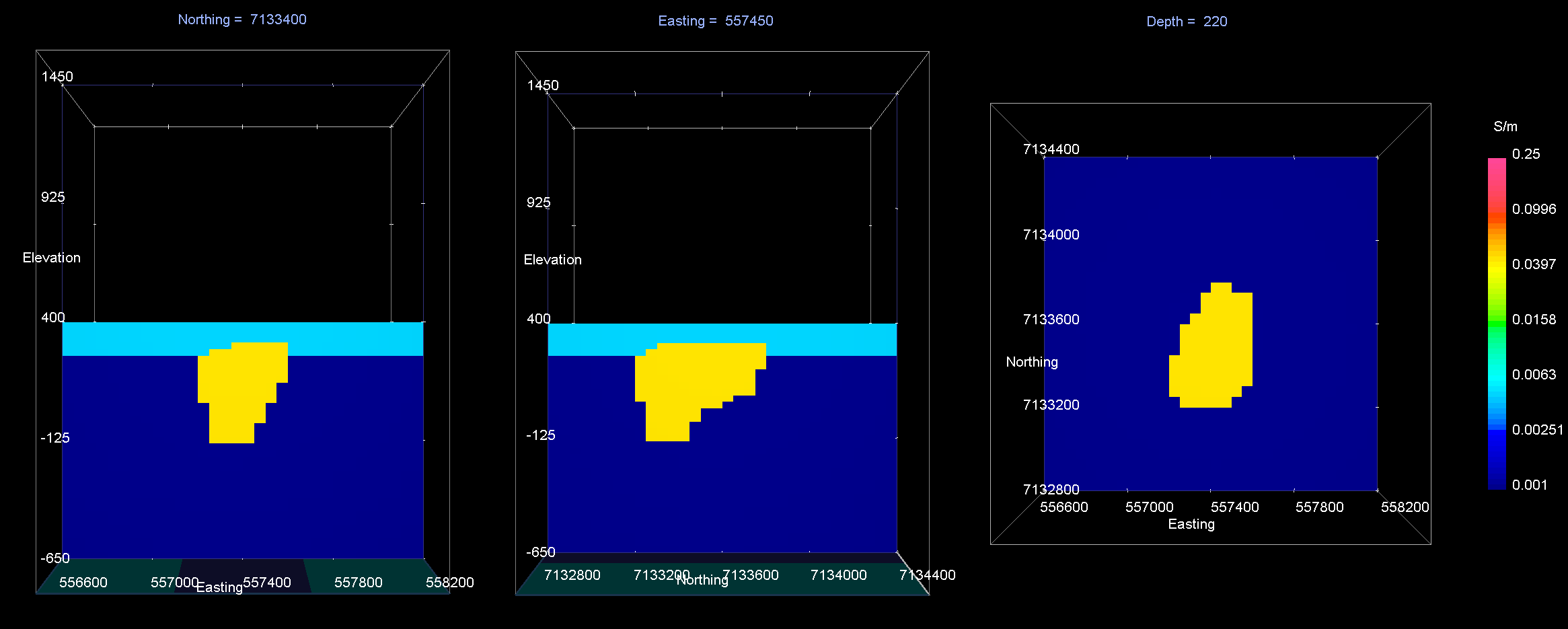True model. EW cross-section (left), NS cross-section (centre), horizontal slice (right).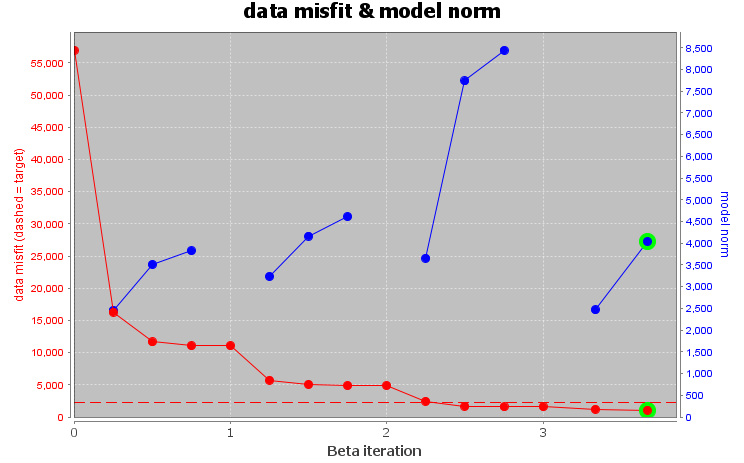Convergence curve shows that inversion reaches target misfit. The model norm is discontinuous because the reference modeled is updated at each iteration.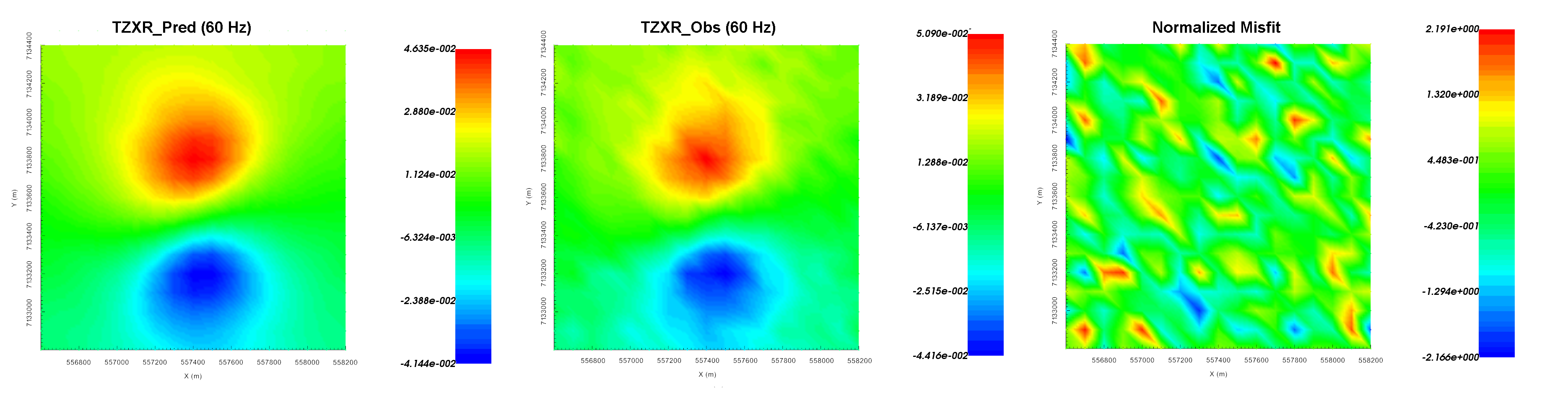Predicted data (left), observed data (centre) and normalized misfit (right) for the real component of $$T_{zx}$$ at 60 Hz.

## 9.6.3.3. E3DMT Version 2¶

Let us now invert the ZTEM data using E3DMT version 2. Unlike version 1, version 2 requires that user define the receiver which measure the fields.

• Click the impedance data object and set receivers from locations. Use the following values:

• Easting width = 2 m

• Northing width = 2 m

• Vertical width = 2 m

• Create E3DMT ver 2 inversion object

• Use edit options to set the inversion parameters

• Basic Tab:
• Select the impedance data

• Set mesh

• Set topography to active cells model

• No background susceptibility

• 1D conductivity of 0.001 S/m (which we inferred from apparent resistivity maps)

• Use Iterative solver unless you have sufficient RAM to use Direct solver.

• Model Options Tab:
• Set Beta cooling schedule to ‘custom by clicking button’. Use beta max = 0.8, beta min = 1e-8 and reduction factor = 0.25

• Set Chi Factor = 1

• alpha S = 1e-10, alpha E = 1, alpha N = 1 and alpha Z = 2.56 (to recover smoothest model and balance gradient terms based on cell dimensions)

• Set the active cells topo as the active model cells

• Set initial model as 0.001 S/m

• Set reference model as 0.001 S/m

• Set role in model objective function to SMOOTH_MOD_DIF

• Click Apply and write files

• Run the inversion

• View convergence

The results of the inversion are shown below. We are unable to recover the moderately conductive overburden as ZTEM data are not sensitive to layered structures. Despite only inverting data at 2 frequencies, we are generally able to recover the shape of the pipe. The maximum conductivity of the recovered structure is notably higher than the true conductivity of the pipe.

The inversion reaches target misfit after 4 iterations, but we have chosen to show the model at iteration 3 (chi factor of 1.005). The model norm is discontinuous because the current model is set to be the reference model for the next beta value. The recovered model is able to reproduce the observed anomaly but underestimates its amplitude.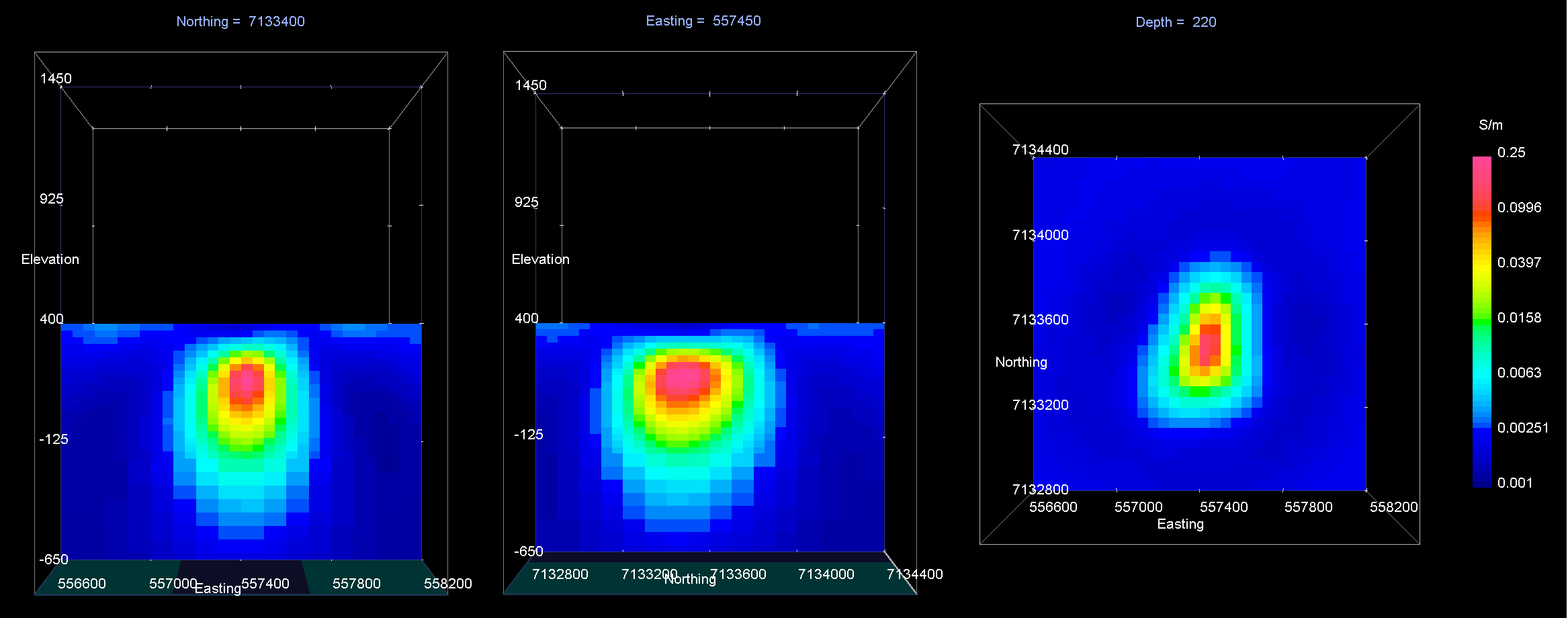Recovered model at iteration 3. EW cross-section (left), NS cross-section (centre), horizontal slice (right).True model. EW cross-section (left), NS cross-section (centre), horizontal slice (right).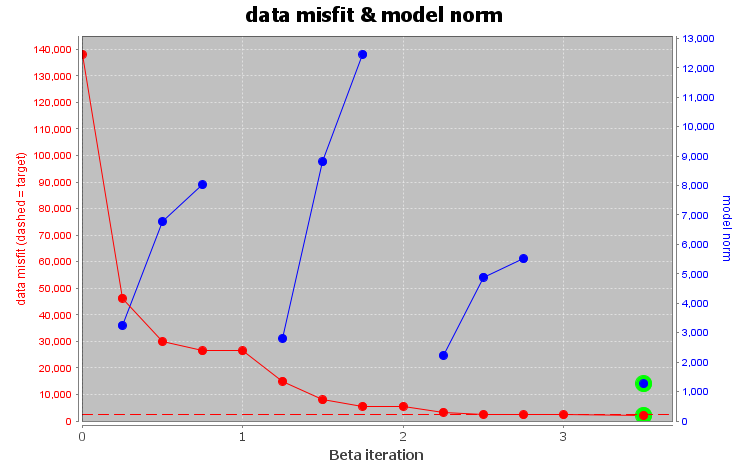Convergence curve shows that inversion reaches target misfit. The model norm is discontinuous because the reference modeled is updated at each iteration.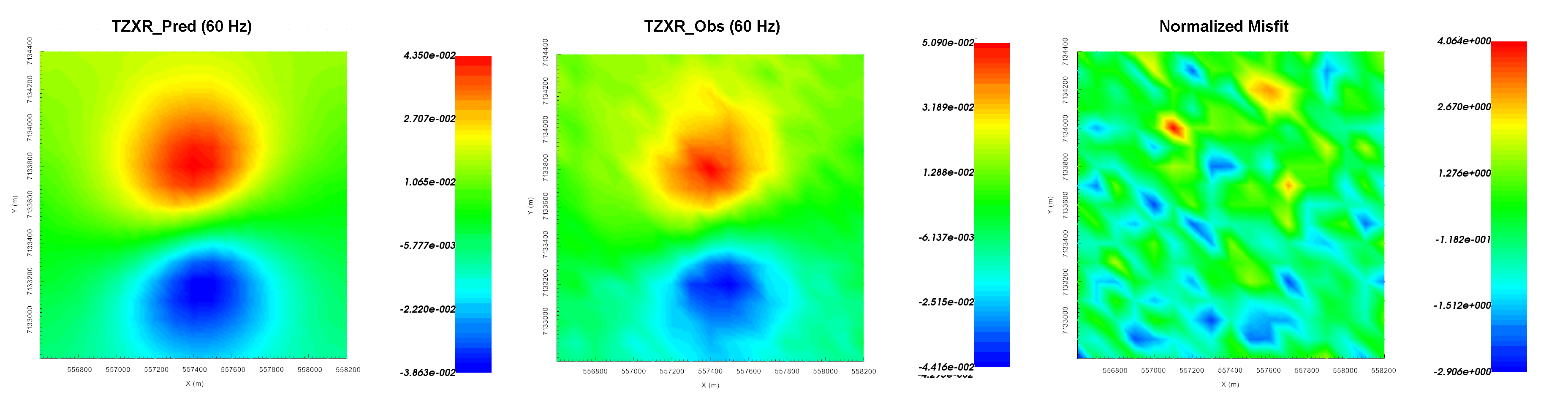Predicted data (left), observed data (centre) and normalized misfit (right) for the real component of $$T_{zx}$$ at 60 Hz.